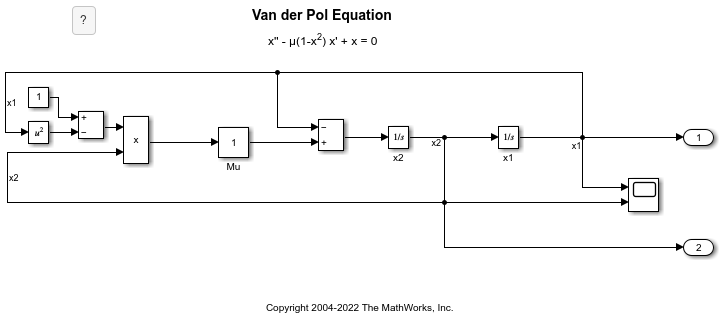## Create and Edit Annotations Programmatically

Annotations are visual elements that you can use to add descriptive notes and callouts to your model. In addition to text-only annotations, you can create annotations that:

• Open websites

• Perform MATLAB® commands

• Display images

• Visually differentiate areas of block diagrams

The following examples show how to programmatically create, edit, and delete annotations.

### Create Annotation Programmatically

Programmatically create, modify, and view an annotation.

Open a new model.

`open_system(new_system)`

Create an annotation with default properties using the `Simulink.Annotation` function.

`a = Simulink.Annotation(gcs,'This is an annotation.');`

After creating the annotation, use dot notation to set property values. For example, apply an 18-point font and light blue background to the annotation.

```a.FontSize = 18; a.BackgroundColor = 'lightBlue';```

To view and briefly highlight the new annotation, use the `view` function.

`view(a)`

### Programmatically Find and Modify Existing Annotations

Programmatically find and modify the properties of an annotation.

Open the `vdp` model.

`vdp`

To find the annotations in the model, use the `find_system` function.

`h = find_system(gcs,'FindAll','on','Type','annotation');`

To identify the annotations, query the text inside the annotations by using the `get_param` function.

`get_param(h,'PlainText')`
```ans = 2x1 cell {'Copyright 2004-2020 The MathWorks, Inc.'} {'van der Pol Equation' } ```

Suppose you want to apply a light blue background color to the `'van der Pol Equation'` annotation.

Get the `Simulink.Annotation` object by specifying the corresponding index of the array.

`a = get_param(h(2),'Object');`

Use dot notation to set the value of the `BackgroundColor` property.

`a.BackgroundColor = 'lightBlue';`

### Delete Annotation

Programmatically delete an annotation.

Open the `vdp` model.

`vdp`

To get the handles for the annotations in the model, use the `find_system` function.

`h = find_system(gcs,'FindAll','on','Type','annotation');`

To identify the annotations, query the text inside the annotations.

`get_param(h,'PlainText')`
```ans = 2x1 cell {'Copyright 2004-2020 The MathWorks, Inc.'} {'van der Pol Equation' } ```

To delete the title of the model (`'van der Pol Equation'`), get the `Simulink.Annotation` object that corresponds to the second handle.

`a = get_param(h(2),'Object');`

Delete the annotation from the model.

`delete(a)`

### Create Annotations That Contain Hyperlinks

For rich-text annotations, you can use HTML formatting to add a hyperlink to text within the annotation.

Open a new model.

`open_system(new_system)`

Create two annotations, moving one of the annotations so that it does not overlap the other.

```a1 = Simulink.Annotation(gcs,'This is an annotation.'); a2 = Simulink.Annotation(gcs,'This is another annotation.'); a2.Position = [0 20 28 34];```

To create a hyperlink in the annotation, set `Interpreter` to `'rich'` and define the hyperlink in the `Text` property.

```a1.Interpreter = 'rich'; a1.Text = 'Go to <a href="www.mathworks.com">www.mathworks.com</a>.';```

You can also embed MATLAB functions in the hyperlink.

```a2.Interpreter = 'rich'; a2.Text = '<a href="matlab:magic(4)">Generate magic square</a>.';```

Add an image to your model, such as a logo, by creating an image-only annotation.

Open a new model and create an annotation in it.

```open_system(new_system) a = Simulink.Annotation(gcs,'This is an annotation.');```

Change the annotation to display only the specified image.

```img = fullfile(matlabroot,'toolbox','matlab','imagesci','peppers.png'); setImage(a,img)```

### Create Area Programmatically

Create an area annotation in a model.

Open the `vdp` model.

`open_system('vdp')`

Create an area that includes some of the blocks in the model.

`add_block('built-in/Area','vdp/This is an area','Position',[120,100,230,200])`### Create and Hide Markup Annotation

To create annotations that can be easily hidden, create markup annotations.

Open a new model.

`open_system(new_system)`

Create two annotations, and move the second annotation so that it does not overlap the first annotation.

```a1 = Simulink.Annotation(gcs,'This is a model annotation.'); a2 = Simulink.Annotation(gcs,'This is a markup annotation.'); a2.Position = [0 20 28 34];```

By default, you create model annotations, which appear in the model.

Change the second annotation to a markup annotation.

`a2.MarkupType = 'markup';`

Configure the current model to hide markup annotations.

`set_param(gcs,'ShowMarkup','off');`

Both annotations remain, despite the markup annotation being hidden.

```ah = find_system(gcs,'FindAll','on','Type','annotation'); at = get_param(ah,'Text')```
```at = 2x1 cell {'This is a markup annotation.'} {'This is a model annotation.' } ```

### Find Annotation Executing Callback Function

If an annotation invoked a currently executing callback function, use the `getCallbackAnnotation` to determine which annotation invoked it. The function returns the corresponding `Annotation` object. This function is also useful if you write a callback function in a separate MATLAB file that contains multiple callback calls.